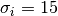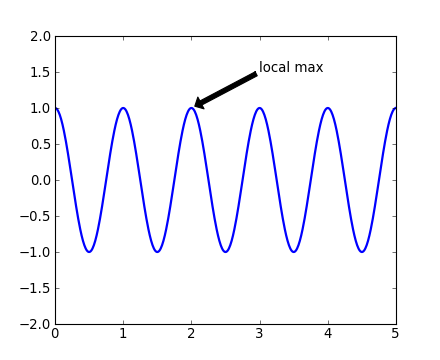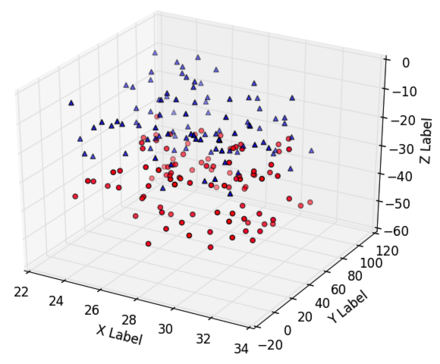# Matplotlib¶

Matplotlib is a Python 2-d and 3-d plotting library which produces publication quality figures in a variety of formats and interactive environments across platforms. Matplotlib can be used in Python scripts, the Python and IPython shell, web application servers, and six graphical user interface toolkits.

## Documentation¶

The matplotlib documentation is extensive and covers all the functionality in detail. The documentation is littered with hundreds of examples showing a plot and the exact source code making the plot:

### Hints on getting from here (an idea) to there (a publishable plot)¶

• Go to the thumbnail gallery and scan the thumbnails to find something similar.
• Googling is unfortunately not the best way to get to the detailed help for particular functions. For example googling “matplotlib errorbar” just gives the home page and the pyplot API docs. The errorbar() function is then not so easy to find.
• Instead use Search and enter the function name. Most of the high-level plotting functions are in the pyplot module and you can find them quickly by searching for pyplot.<function>, e.g. pyplot.errorbar.
• When you are ready to put your plot into a paper for publication see the page on Publication-quality plots.

## Plotting 1-d data¶

The matplotlib tutorial on Pyplot (Copyright (c) 2002-2009 John D. Hunter; All Rights Reserved and license) is an excellent introduction to basic 1-d plotting. The content below has been adapted from the pyplot tutorial source with some changes and the addition of exercises.

### Basic plots¶

So let’s get started with plotting using a standard startup idiom that will work for both interactive and scripted plotting. In this case we are working interactively so fire up ipython in the usual way with the standard imports for numpy and matplotlib:

## Using mathematical expressions in text¶

matplotlib accepts TeX equation expressions in any text expression. For example to write the expressionin the title, you can write a TeX expression surrounded by dollar signs:

plt.title(r'$\sigma_i=15$')


The r preceeding the title string is important – it signifies that the string is a raw string and not to treate backslashes as python escapes. matplotlib has a built-in TeX expression parser and layout engine, and ships its own math fonts – for details see the mathtext-tutorial. Thus you can use mathematical text across platforms without requiring a TeX installation. For those who have LaTeX and dvipng installed, you can also use LaTeX to format your text and incorporate the output directly into your display figures or saved postscript – see the usetex-tutorial.

## Annotating text¶

The uses of the basic text() command above place text at an arbitrary position on the Axes. A common use case of text is to annotate some feature of the plot, and the annotate() method provides helper functionality to make annotations easy. In an annotation, there are two points to consider: the location being annotated represented by the argument xy and the location of the text xytext. Both of these arguments are (x,y) tuples:

plt.clf()
t = np.arange(0.0, 5.0, 0.01)
s = np.cos(2*pi*t)
lines = plt.plot(t, s, lw=2)

plt.annotate('local max', xy=(2, 1), xytext=(3, 1.5),
arrowprops=dict(facecolor='black', shrink=0.05))
plt.ylim(-2,2)In this basic example, both the xy (arrow tip) and xytext locations (text location) are in data coordinates. There are a variety of other coordinate systems one can choose – see the Annotations introduction for more details and links to examples.

## Plotting 2-d data¶

A deeper tutorial on plotting 2-d image data will have to wait for another day:

• For simple cases it is straightforward and everything you need to know is in the image tutorial
• For making publication quality images for astronomy you should be using APLpy.

## Plotting 3-d data¶

Matplotlib supports plotting 3-d data through the mpl_toolkits.mplot3d module. This is a somewhat specialized functionality but it’s worth quickly looking at an example of the 3-d viewer that is available:

from mpl_toolkits.mplot3d import Axes3D

def randrange(n, vmin, vmax):
return (vmax-vmin)*np.random.rand(n) + vmin

fig = plt.figure()
n = 100
for c, m, zl, zh in [('r', 'o', -50, -25), ('b', '^', -30, -5)]:
xs = randrange(n, 23, 32)
ys = randrange(n, 0, 100)
zs = randrange(n, zl, zh)
ax.scatter(xs, ys, zs, c=c, marker=m)

ax.set_xlabel('X Label')
ax.set_ylabel('Y Label')
ax.set_zlabel('Z Label')## Appendix: Pylab and Pyplot and NumPy¶

You may see examples that use the pylab mode of IPython by using ipython --pylab or the %pylab magic function. Let’s demystify what’s happening in this case and clarify the relationship between pylab and pyplot.

matplotlib.pyplot is a collection of command style functions that make matplotlib work like MATLAB. This is just a package module that you can import:

import matplotlib.pyplot
print(sorted(dir(matplotlib.pyplot)))


Likewise pylab is also a module provided by matplotlib that you can import:

import pylab


This module is a thin wrapper around matplotlib.pylab which pulls in:

• Everything in matplotlib.pyplot
• All top-level functions numpy, numpy.fft, numpy.random, numpy.linalg
• A selection of other useful functions and modules from matplotlib

There is no magic, and to see for yourself do

import matplotlib.pylab
matplotlib.pylab??       # prints the source code!!


When you do ipython --pylab it (essentially) just does:

from pylab import *


There is one technical detail about GUI event loops and plot window interaction which makes it useful to use --pylab even if you are not directly using all the top-level functions like plot() that get imported.

In a lot of documentation examples you will see code like:

import matplotlib.pyplot as plt  # set plt as alias for matplotlib.pyplot
plt.plot([1,2], [3,4])


Now you should understand this is the same plot() function that you get in Pylab.

See Matplotlib, pylab, and pyplot: how are they related? for a more discussion on the topic.